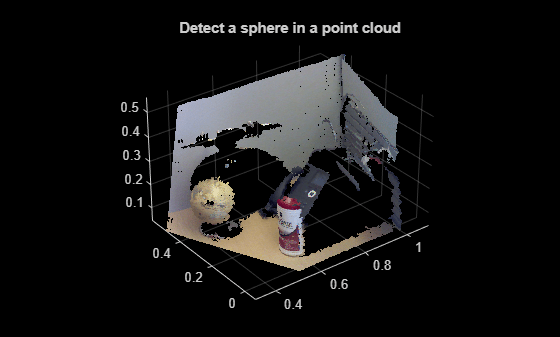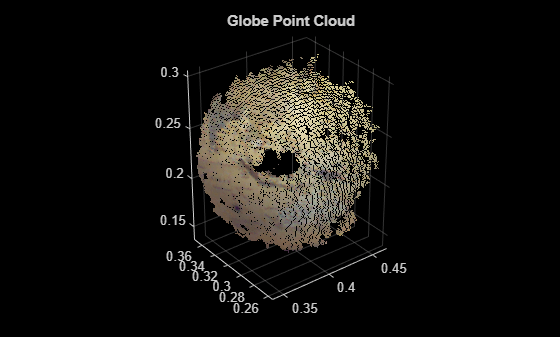# sphereModel.plot

Plot sphere in a figure window

## Syntax

``plot(model)``
``plot(model,'Parent',ax)``

## Description

````H` = `plot(model)` plots a sphere in the current figure. `H` is the handle to `surf`, a 3-D shaded surface plot.```
````H` = `plot(model,'Parent',ax)` additionally allows you to specify an output axes.```

## Input Arguments

collapse all

Parametric sphere model returned by `sphereModel`.

Output axes, specified as the comma-separated pair of `'Parent'` and the current axes for displaying the visualization.

## Examples

collapse all

`load('object3d.mat');`

Display point cloud.

```figure pcshow(ptCloud) xlabel('X(m)') ylabel('Y(m)') zlabel('Z(m)') title('Detect a sphere in a point cloud')```Set the maximum point-to-sphere distance (1cm), for sphere fitting.

`maxDistance = 0.01;`

Set the region of interest to constrain the search.

```roi = [-inf, 0.5; 0.2, 0.4; 0.1, inf]; sampleIndices = findPointsInROI(ptCloud, roi);```

Detect the globe in the point cloud and extract it.

`model = pcfitsphere(ptCloud, maxDistance, 'SampleIndices', sampleIndices);`

Plot the sphere.

```hold on plot(model)```